Learn how »

# Mathematica Basics

This screencast helps you to get started using Mathematica by introducing some of the most basic concepts, including entering input, understanding the anatomy of functions, working with data and matrix operations, and finding functions.Learn the basics of Mathematica and get started using it in your day-to-day work. This video will show you how to create and organize content in your notebook, use free-form ...See how Wolfram Notebooks are not just a great working environmentbut can be used to publish work, collaborate with colleagues or get grades and feedback from your teacher.Wolfram Notebooks are the modern environment for technical workflows. These powerful and interactive documents for computation, programming, generating reports and creating presentations produce immediate results and reach your audience on ...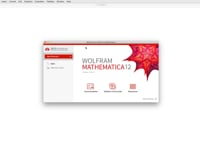This Hands-on Start to Mathematica (Version 12) tutorial screencast provides step-by-step instruction to get you started with Mathematica. During this video, you'll learn how to enter queries through free-form input and ...This film discusses the techniques, and outlines the vision of the future computerization of pure mathematics through interviews and talk segments from renowned mathematicians, meta-mathematicians, computational mathematicians, and theorem provers.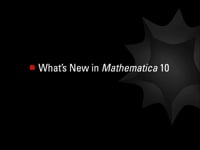With over 700 new functions and cloud integration, Mathematica 10 introduces a host of new areas. See what's new in this videofrom machine learning and computational geometry to geographic computation and ...Hands-on Start to Mathematica is an 8-part tutorial screencast series that provides step-by-step instruction to get you started with Mathematica. In this first video of the series, you'll learn how ...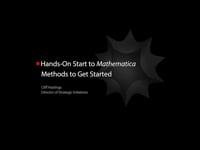Hands-on Start to Mathematica is an 8-part tutorial screencast series that provides step-by-step instruction to get you started with Mathematica. In this second video of the series, you'll learn how ...Hands-on Start to Mathematica is an 8-part tutorial screencast series that provides step-by-step instruction to get you started with Mathematica. In this third video of the series, you'll discover how ...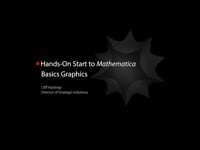Hands-on Start to Mathematica is an 8-part tutorial screencast series that provides step-by-step instruction to get you started with Mathematica. In this fourth video of the series, you'll learn how ...Hands-on Start to Mathematica is an 8-part tutorial screencast series that provides step-by-step instruction to get you started with Mathematica. In this fifth video of the series, you'll learn how to take anything--- a calculation, a graphic, a piece of text--- and turn it into an interactive model that you can use ...Hands-on Start to Mathematica is an 8-part tutorial screencast series that provides step-by-step instruction to get you started with Mathematica. In this sixth video of the series, you'll get a ...Hands-on Start to Mathematica is an 8-part tutorial screencast series that provides step-by-step instruction to get you started with Mathematica. In this seventh video of the series, you'll learn how ...Hands-on Start to Mathematica is an 8-part tutorial screencast series that provides step-by-step instruction to get you started with Mathematica. In this last video of the series, you'll get a ...See why Mathematica is the complete homework and research software to calculate and analyze, learn and explore, visualize and illustrate, and document and publish.Wolfram technologies are fueling innovation within industry and redefining the landscape for classroom learning. Discover how at a Wolfram Technology Seminar.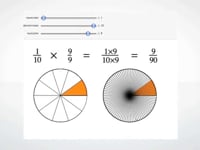Take a quick tour of Mathematica and see what makes it a complete environment for education and academic research.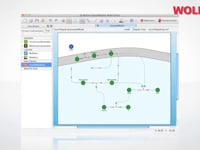See how Mathematica, Wolfram SystemModeler, Wolfram|Alpha, and other Wolfram technologies are advancing biosciences.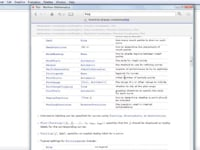Mathematica provides several convenient ways to find information about functions. In addition to searching the documentation or navigating the guide pages, you can access documentation on functions directly from within ...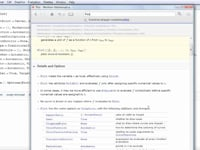Mathematica gives you control over the behavior of most functions by providing options that you can customize. Learn more about finding available options for functions in this "How to" screencast.